# Camera Structure

## 1. Lens and Focal Point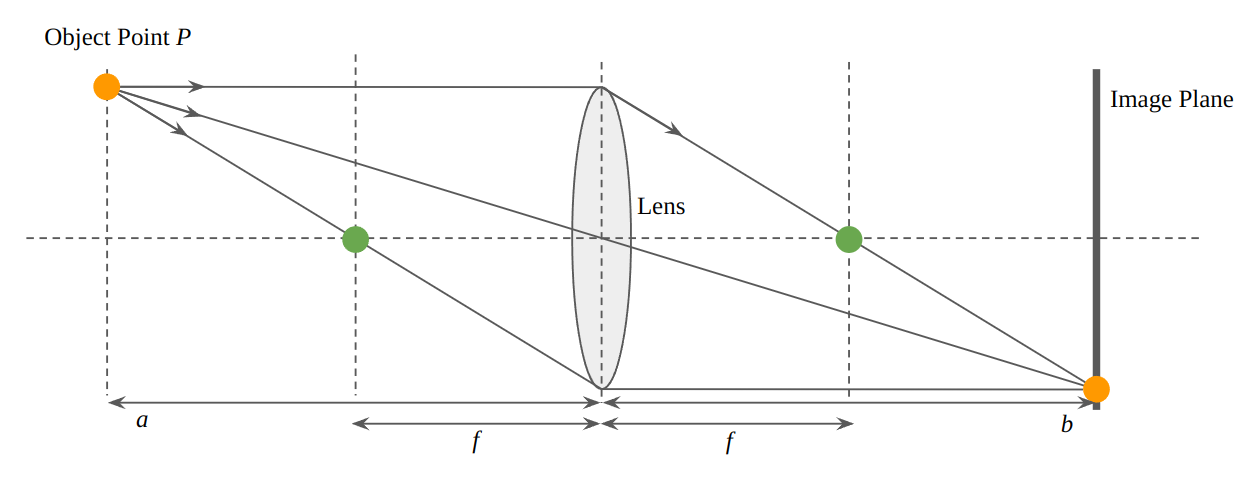• The light through the center of the lens is not refracted.
• The light parallel with the axis of the lens passes through the focal point after refracted.
• The light through the focal point in front of the lens is parallel with the axis of the lens after refracted.
• $P$ is most sharp when it is projected on image plane, and $\frac{1}{a} + \frac{1}{b} = \frac{1}{f}$.
• The longer $f$ is, the further objects the camera can take like a telephoto lens.
• The shorter $f$ is, the wider range the camera can take like a wide-angle lens.

## 2. Focal Length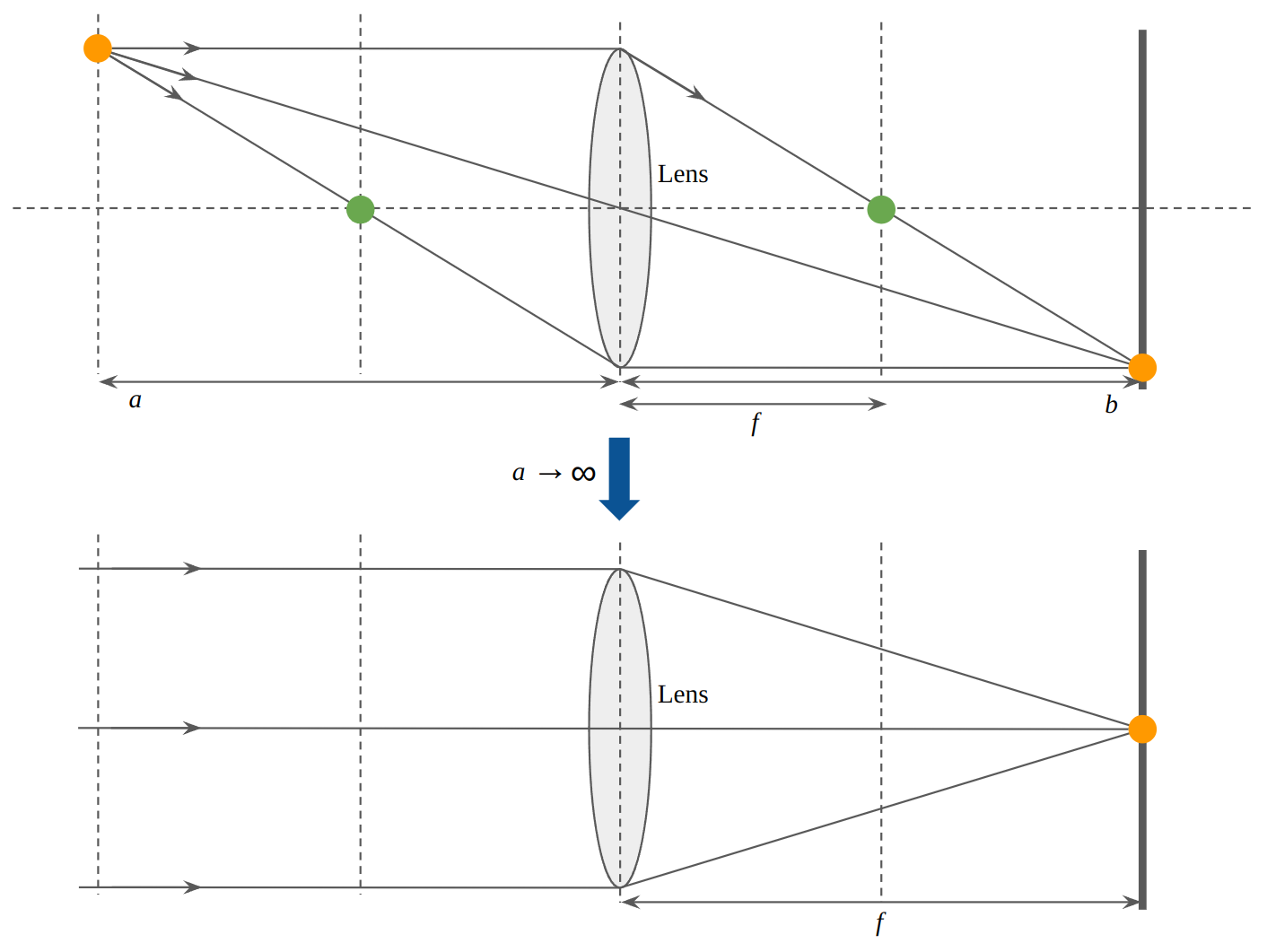• The focal length of the virtual camera in computer graphics is $f$ when $a = \infty$. It implies $b = f$.
• The field of view $\theta = 2 tan^{-1}\frac{L}{2f}$ where $L$ is one of $W$, $H$, and $D$.
• The longer $f$ is, the field of view is wider.
• The shorter $f$ is, the field of view is narrower.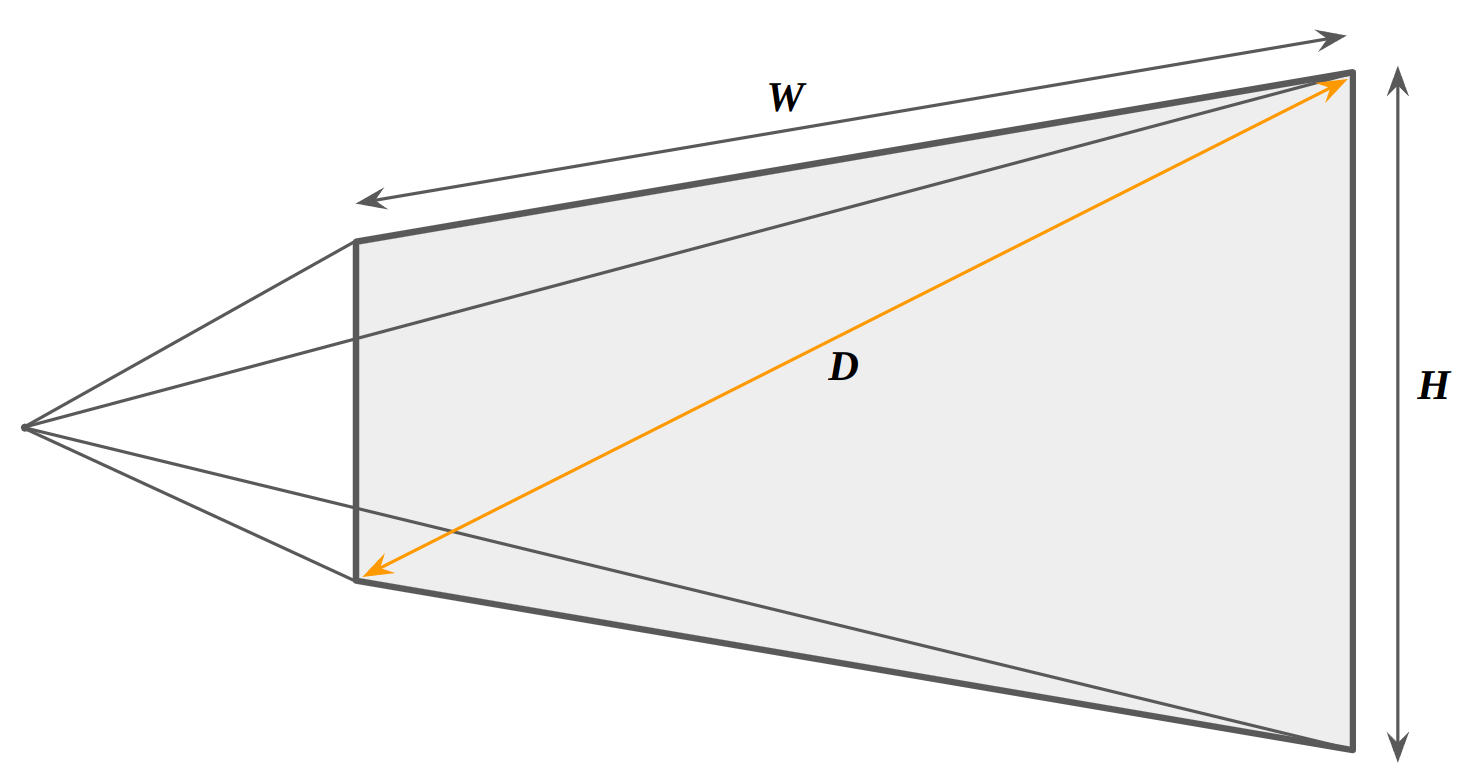## 3. Shutter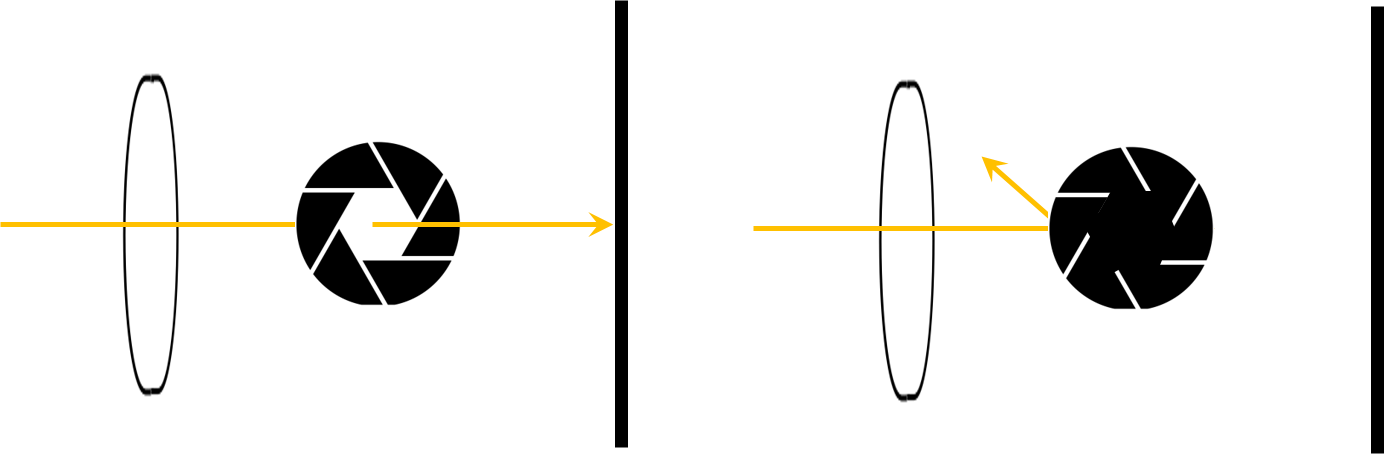• When the light arrive at the image plane, the pixel color is depend on amount of light or color.
• Amount of light is proportional to how long the camera shutter is open.
• Let the shutter opening time be $t$, then a bright image will be created as $t$ is long and a dark image as $t$ is short.

## 4. Aperture

• The aperture controls amount of light by adjusting entrance of light.
• The status of the aperture is denoted by $F$ value, which means that if the radius of the aperture is reduced to $\frac{1}{\sqrt{2}}$ of itself, its area is reduced to half.
• $F$ is presented per $\sqrt{2} \approx 1.4$ such as $1, 1.4, 2, 2.8, 4, 5.6, 8, 11, 16, 22 ...$. The higher $F$ is, the smaller the area of the aperture is by its half.

## 5. Pinhole Camera Model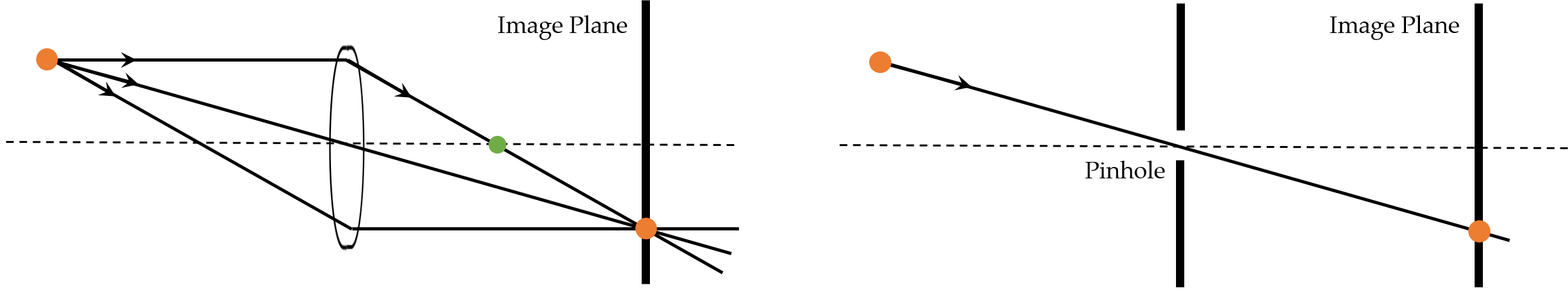• In general, the image is focused when the light from diverse directions meet at one point on the image plane. However, the image is always focused everywhere in pinhole camera model since the light from the only one direction can arrive at the image plane.

## 6. Bokeh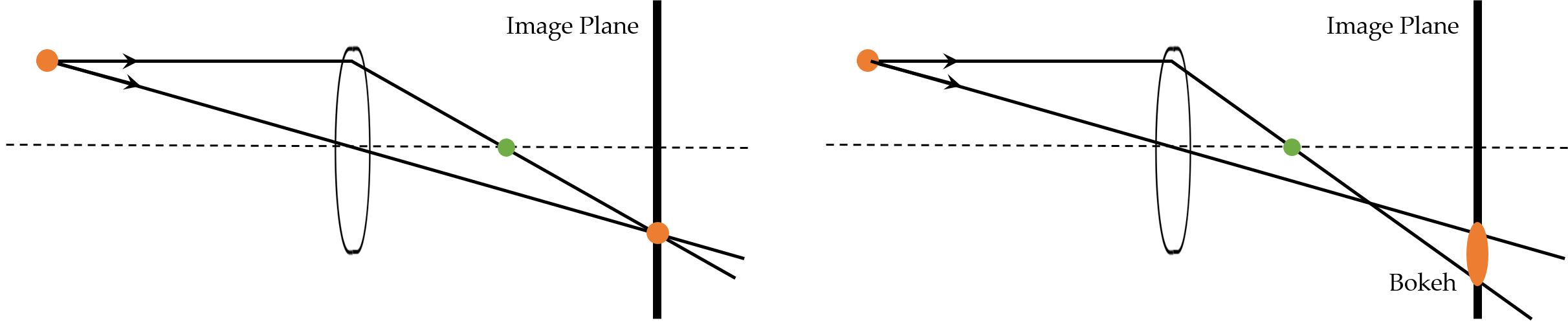*Bokeh is a kind of blurring or out-of-focus which is the technique for the aesthetic purpose. In other words, It happens when a obejct point is not projected on the image plane as one pixel point.

## 7. Depth of Field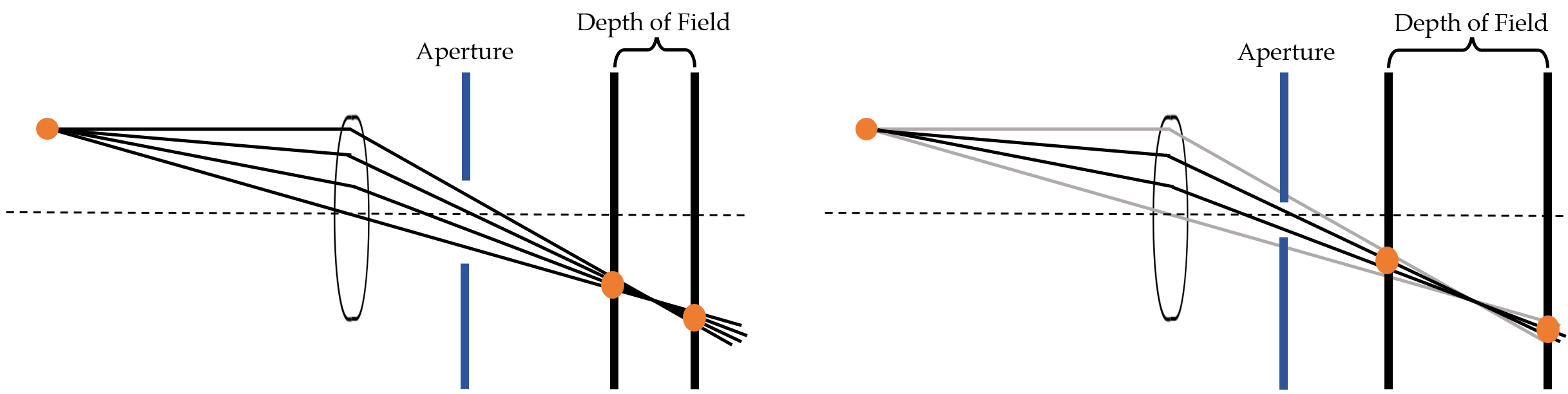• In fact, the image is focused in some range which is called depth of field. In other words, it is a range of bokeh which can be considered that the image is focused.
• As the aperture of the camera is closed, the depth of field gets deeper.
• For a pinhole camera, it is considerd that the aperture is almost closed. That is why a pinhole camera is focused everywhere.

## Reference

 카츠라 요스케 , 요츠쿠라 타츠오 , 마크 살바티, 테크니컬 아티스트를 위한 최고의 교과서: 내공 있는 CG 아티스트로 이끌어주는 체계적인 입문서, 2014